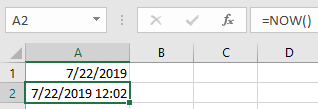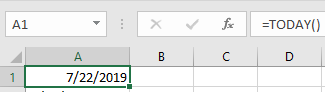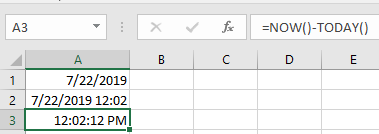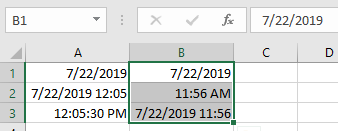# How to use the NOW function in ExcelIn this article, we will learn How to use the NOW function in Excel.

Today's date and time value in Excel

In Excel, If you need to use today's date and time together without any trouble, you just need to use this function. For Example working with attendees for the employee. You cannot afford to have human error while doing so. Let's learn how to use the easiest function in Excel. NOW function syntax and is explained using an example

NOW Function in Excel

NOW function is a date and time function which returns the current date and time together in a cell in the date time format. NOW function is a built-in dynamic function to get current Date and Time in one cell.

NOW Function Syntax :

 =NOW()

For static use of the function use the shortcut key to perform is Ctrl + Shift + ; (semi - colon)

TODAY function is a built-in function to get Today’s Date or Current Date in Excel.

Syntax:

 =TODAY()

For static use of the function use the shortcut key to perform is Ctrl + ; (semi - colon)

NOW function Example :

All of these might be confusing to understand. Let's understand how to use the function using an example. Here are some snapshots to see how it works

NOW function does not take any parameters. It is used with parentheses.TODAY function used in the A1 cell. TODAY function like NOW function does not take any parameters. It is used with parentheses.NOW & TODAY function to get the Current TimeShortcut keys to perform the same function as above

In the B1 cell use Shortcut key to get current date is Ctrl + ; (semi-colon)

In the B2 cell use shortcut key to get current time is Ctrl + Shift + ; (semi-colon)In the B3 cell use Ctrl + ; (semi-colon) and After single space use Ctrl +Shift + ; (semi-colon) to get both date & time in the same cell.

Note: Dynamic function returns result whenever updated or refreshed whereas static function return results remain constant until & unless edited.

Here are all the observational notes using the NOW function in Excel
Notes :

1. Excel stores dates as serial numbers and is used in calculation by the function. So it’s recommended to use dates as cell reference or using the DATE function instead of giving direct argument to the function. Check the validation of date values if the function returns #VALUE! Error.
2. DAYS function can also subtract the two given numbers. Careful using the function
3. The date in excel must be in valid format
4. Unit argument in DATEDIF function can be given as cell reference or directly to the function in double quotes.
5. Start_date must be before the end_date or else the function will give #NUM! error
6. The function gives #VALUE! Error if the date is not a valid date.

Hope this article about How to use the NOW function in Excel is explanatory. Find more articles on calculating values and related Excel formulas here. If you liked our blogs, share it with your friends on Facebook. And also you can follow us on Twitter and Facebook. We would love to hear from you, do let us know how we can improve, complement or innovate our work and make it better for you. Write to us at info@exceltip.com.

Related Articles :

How to Convert date to text in Excel : In this article we learned how to convert text into date, but how do you convert an excel date into text. To convert an excel date into text we have a few techniques.

Excel converts decimal Seconds into time format : As we know that time in excel is treated as numbers. Hours, Minutes, and Seconds are treated as decimal numbers. So when we have seconds as numbers, how do we convert into time format? This article got it covered.

How to use the NETWORKDAYS function in Excel : Returns the working days between the two given dates using the NETWORKDAYS function.

How to use the WORKDAYS function in Excel : Returns a workday date value after days added to the start_date using the WORKDAYS function in Excel.

Count holidays between dates in Excel : count non working days between the two given dates using the DATEDIF and NETWORKDAYS function in Excel.

Extract days between dates ignoring years in Excel : count the days between the two dates using different functions and mathematical operation in Excel

Count Birth Dates By Month in Excel : count the number of dates lying in a particular month using the SUMPRODUCT and MONTH function in Excel.

SUM price by weekdays in Excel : sum price values corresponding to the number of dates in a particular weekday using the SUMPRODUCT and WEEKDAY function in Excel.

Popular Articles :

How to use the IF Function in Excel : The IF statement in Excel checks the condition and returns a specific value if the condition is TRUE or returns another specific value if FALSE.

How to use the VLOOKUP Function in Excel : This is one of the most used and popular functions of excel that is used to lookup value from different ranges and sheets.

How to use the SUMIF Function in Excel : This is another dashboard essential function. This helps you sum up values on specific conditions.

How to use the COUNTIF Function in Excel : Count values with conditions using this amazing function. You don't need to filter your data to count specific values. Countif function is essential to prepare your dashboard.

Terms and Conditions of use

The applications/code on this site are distributed as is and without warranties or liability. In no event shall the owner of the copyrights, or the authors of the applications/code be liable for any loss of profit, any problems or any damage resulting from the use or evaluation of the applications/code.